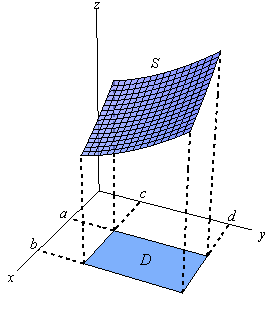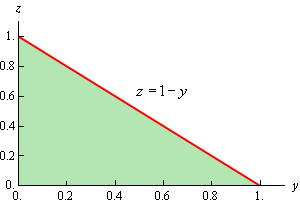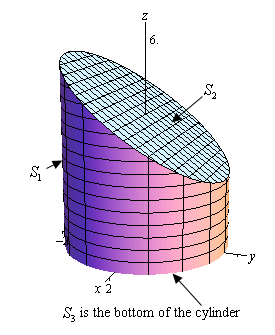Paul's Online Notes
Home / Calculus III / Surface Integrals / Surface Integrals
Show Mobile Notice Show All Notes Hide All Notes
Mobile Notice
You appear to be on a device with a "narrow" screen width (i.e. you are probably on a mobile phone). Due to the nature of the mathematics on this site it is best views in landscape mode. If your device is not in landscape mode many of the equations will run off the side of your device (should be able to scroll to see them) and some of the menu items will be cut off due to the narrow screen width.

### Section 17.3 : Surface Integrals

It is now time to think about integrating functions over some surface, $$S$$, in three-dimensional space. Let’s start off with a sketch of the surface $$S$$ since the notation can get a little confusing once we get into it. Here is a sketch of some surface $$S$$.The region $$S$$ will lie above (in this case) some region $$D$$ that lies in the $$xy$$-plane. We used a rectangle here, but it doesn’t have to be of course. Also note that we could just as easily looked at a surface $$S$$ that was in front of some region $$D$$ in the $$yz­$$-plane or the $$xz$$-plane. Do not get so locked into the $$xy$$-plane that you can’t do problems that have regions in the other two planes.

Now, how we evaluate the surface integral will depend upon how the surface is given to us. There are essentially two separate methods here, although as we will see they are really the same.

First, let’s look at the surface integral in which the surface $$S$$ is given by $$z = g\left( {x,y} \right)$$. In this case the surface integral is,

$\iint\limits_{S}{{f\left( {x,y,z} \right)\,dS}} = \iint\limits_{D}{{f\left( {x,y,g\left( {x,y} \right)} \right)\sqrt {{{\left( {\frac{{\partial g}}{{\partial x}}} \right)}^2} + {{\left( {\frac{{\partial g}}{{\partial y}}} \right)}^2} + 1} \,dA}}$

Now, we need to be careful here as both of these look like standard double integrals. In fact the integral on the right is a standard double integral. The integral on the left however is a surface integral. The way to tell them apart is by looking at the differentials. The surface integral will have a $$dS$$ while the standard double integral will have a $$dA$$.

In order to evaluate a surface integral we will substitute the equation of the surface in for $$z$$ in the integrand and then add on the often messy square root. After that the integral is a standard double integral and by this point we should be able to deal with that.

Note as well that there are similar formulas for surfaces given by $$y = g\left( {x,z} \right)$$ (with $$D$$ in the $$xz$$-plane) and $$x = g\left( {y,z} \right)$$ (with $$D$$ in the $$yz$$-plane). We will see one of these formulas in the examples and we’ll leave the other to you to write down.

The second method for evaluating a surface integral is for those surfaces that are given by the parameterization,

$\vec r\left( {u,v} \right) = x\left( {u,v} \right)\vec i + y\left( {u,v} \right)\vec j + z\left( {u,v} \right)\vec k$

In these cases the surface integral is,

$\iint\limits_{S}{{f\left( {x,y,z} \right)\,dS}} = \iint\limits_{D}{{f\left( {\vec r\left( {u,v} \right)} \right)\left\| {{{\vec r}_u} \times {{\vec r}_v}} \right\|\,dA}}$

where $$D$$ is the range of the parameters that trace out the surface $$S$$.

Before we work some examples let’s notice that since we can parameterize a surface given by $$z = g\left( {x,y} \right)$$ as,

$\vec r\left( {x,y} \right) = x\vec i + y\vec j + g\left( {x,y} \right)\vec k$

we can always use this form for these kinds of surfaces as well. In fact, it can be shown that,

$\left\| {{{\vec r}_x} \times {{\vec r}_y}} \right\|\, = \sqrt {{{\left( {\frac{{\partial g}}{{\partial x}}} \right)}^2} + {{\left( {\frac{{\partial g}}{{\partial y}}} \right)}^2} + 1}$

for these kinds of surfaces. You might want to verify this for the practice of computing these cross products.

Let’s work some examples.

Example 1 Evaluate $$\displaystyle \iint\limits_{S}{{6xy\,dS}}$$ where $$S$$ is the portion of the plane $$x + y + z = 1$$ that lies in the 1st octant and is in front of the $$yz$$-plane.
Show Solution

Okay, since we are looking for the portion of the plane that lies in front of the $$yz$$-plane we are going to need to write the equation of the surface in the form $$x = g\left( {y,z} \right)$$. This is easy enough to do.

$x = 1 - y - z$

Next, we need to determine just what $$D$$ is. Here is a sketch of the surface $$S$$.Here is a sketch of the region $$D$$.Notice that the axes are labeled differently than we are used to seeing in the sketch of $$D$$. This was to keep the sketch consistent with the sketch of the surface. We arrived at the equation of the hypotenuse by setting $$x$$ equal to zero in the equation of the plane and solving for $$z$$. Here are the ranges for $$y$$ and $$z$$.

$0 \le y \le 1\hspace{0.25in}0 \le z \le 1 - y$

Now, because the surface is not in the form $$z = g\left( {x,y} \right)$$ we can’t use the formula above. However, as noted above we can modify this formula to get one that will work for us. Here it is,

$\iint\limits_{S}{{f\left( {x,y,z} \right)\,dS}} = \iint\limits_{D}{{f\left( {g\left( {y,z} \right),y,z} \right)\sqrt {1 + {{\left( {\frac{{\partial g}}{{\partial y}}} \right)}^2} + {{\left( {\frac{{\partial g}}{{\partial z}}} \right)}^2}} \,dA}}$

The changes made to the formula should be the somewhat obvious changes. So, let’s do the integral.

$\iint\limits_{S}{{6xy\,dS}} = \iint\limits_{D}{{6\left( {1 - y - z} \right)y\sqrt {1 + {{\left( { - 1} \right)}^2} + {{\left( { - 1} \right)}^2}} \,dA}}$

Notice that we plugged in the equation of the plane for the x in the integrand. At this point we’ve got a fairly simple double integral to do. Here is that work.

\begin{align*}\iint\limits_{S}{{6xy\,dS}} & = \sqrt 3 \iint\limits_{D}{{6\left( {y - {y^2} - zy} \right)\,dA}}\\ & = 6\sqrt 3 \int_{{\,0}}^{{\,1}}{{\int_{{\,0}}^{{\,1 - y}}{{y - {y^2} - zy\,dz}}\,dy}}\\ & = 6\sqrt 3 \int_{{\,0}}^{{\,1}}{{\left. {\left( {yz - z{y^2} - \frac{1}{2}{z^2}y} \right)} \right|_0^{1 - y}\,dy}}\\ & = 6\sqrt 3 \int_{{\,0}}^{{\,1}}{{\frac{1}{2}y - {y^2} + \frac{1}{2}{y^3}\,dy}}\\ & = 6\sqrt 3 \left. {\left( {\frac{1}{4}{y^2} - \frac{1}{3}{y^3} + \frac{1}{8}{y^4}} \right)} \right|_0^1 = \frac{{\sqrt 3 }}{4}\end{align*}
Example 2 Evaluate $$\displaystyle \iint\limits_{S}{{z\,dS}}$$ where $$S$$ is the upper half of a sphere of radius 2.
Show Solution

We gave the parameterization of a sphere in the previous section. Here is the parameterization for this sphere.

$\vec r\left( {\theta ,\varphi } \right) = 2\sin \varphi \cos \theta \,\vec i + 2\sin \varphi \sin \theta \,\vec j + 2\cos \varphi \,\vec k$

Since we are working on the upper half of the sphere here are the limits on the parameters.

$0 \le \theta \le 2\pi \hspace{0.5in}0 \le \varphi \le \frac{\pi }{2}$

Next, we need to determine $${\vec r_\theta } \times {\vec r_\varphi }$$. Here are the two individual vectors.

\begin{align*}{{\vec r}_\theta }\left( {\theta ,\varphi } \right) & = - 2\sin \varphi \sin \theta \,\vec i + 2\sin \varphi \cos \theta \,\vec j\\ {{\vec r}_\varphi }\left( {\theta ,\varphi } \right) & = 2\cos \varphi \cos \theta \,\vec i + 2\cos \varphi \sin \theta \,\vec j - 2\sin \varphi \,\vec k\end{align*}

Now let’s take the cross product.

\begin{align*}{{\vec r}_\theta } \times {{\vec r}_\varphi } & = \left| {\begin{array}{*{20}{c}}{\vec i}&{\vec j}&{\vec k}\\{ - 2\sin \varphi \sin \theta }&{2\sin \varphi \cos \theta }&0\\{2\cos \varphi \cos \theta }&{2\cos \varphi \sin \theta }&{ - 2\sin \varphi }\end{array}} \right|\\ & = - 4{\sin ^2}\varphi \cos \theta \,\vec i - 4\sin \varphi \cos \varphi {\sin ^2}\theta \,\vec k - 4{\sin ^2}\varphi \sin \theta \,\vec j - 4\sin \varphi \cos \varphi {\cos ^2}\theta \,\vec k\\ & = - 4{\sin ^2}\varphi \cos \theta \,\vec i - 4{\sin ^2}\varphi \sin \theta \,\vec j - 4\sin \varphi \cos \varphi \left( {{{\sin }^2}\theta \, + {{\cos }^2}\theta } \right)\vec k\\ & = - 4{\sin ^2}\varphi \cos \theta \,\vec i - 4{\sin ^2}\varphi \sin \theta \,\vec j - 4\sin \varphi \cos \varphi \,\vec k\end{align*}

Finally, we need the magnitude of this,

\begin{align*}\left\| {{{\vec r}_\theta } \times {{\vec r}_\varphi }} \right\| & = \sqrt {16{{\sin }^4}\varphi {{\cos }^2}\theta + 16{{\sin }^4}\varphi {{\sin }^2}\theta + 16{{\sin }^2}\varphi {{\cos }^2}\varphi } \\ & = \sqrt {16{{\sin }^4}\varphi \left( {{{\cos }^2}\theta + {{\sin }^2}\theta } \right) + 16{{\sin }^2}\varphi {{\cos }^2}\varphi } \\ & = \sqrt {16{{\sin }^2}\varphi \left( {{{\sin }^2}\varphi + {{\cos }^2}\varphi } \right)} \\ & = 4\sqrt {{{\sin }^2}\varphi } \\ & = 4\left| {\sin \varphi } \right|\\ & = 4\sin \varphi \end{align*}

We can drop the absolute value bars in the sine because sine is positive in the range of $$\varphi$$ that we are working with. The surface integral is then,

$\iint\limits_{S}{{z\,dS}} = \iint\limits_{D}{{2\cos \varphi \left( {4\sin \varphi } \right)\,dA}}$

Don’t forget that we need to plug in for $$x$$, $$y$$ and/or $$z$$ in these as well, although in this case we just needed to plug in $$z$$. Here is the evaluation for the double integral.

\begin{align*}\iint\limits_{S}{{z\,dS}} & = \int_{{\,0}}^{{\,2\pi }}{{\int_{{\,0}}^{{\,\frac{\pi }{2}}}{{4\sin \left( {2\varphi } \right)\,d\varphi }}\,d\theta }}\\ & = \int_{{\,0}}^{{\,2\pi }}{{\left. {\left( { - 2\cos \left( {2\varphi } \right)} \right)} \right|_0^{\frac{\pi }{2}}\,d\theta }}\\ & = \int_{{\,0}}^{{\,2\pi }}{{4\,d\theta }}\\ & = 8\pi \end{align*}
Example 3 Evaluate $$\displaystyle \iint\limits_{S}{{y\,dS}}$$ where $$S$$ is the portion of the cylinder $${x^2} + {y^2} = 3$$ that lies between $$z = 0$$ and $$z = 6$$.
Show Solution

We parameterized up a cylinder in the previous section. Here is the parameterization of this cylinder.

$\vec r\left( {z,\theta } \right) = \sqrt 3 \cos \theta \,\vec i + \sqrt 3 \sin \theta \,\vec j + z\,\vec k$

The ranges of the parameters are,

$0 \le z \le 6\hspace{0.25in}0 \le \theta \le 2\pi$

Now we need $${\vec r_z} \times {\vec r_\theta }$$. Here are the two vectors.

\begin{align*}{{\vec r}_z}\left( {z,\theta } \right) & = \,\vec k\\ {{\vec r}_\theta }\left( {z,\theta } \right) & = - \sqrt 3 \sin \theta \,\vec i + \sqrt 3 \cos \theta \,\vec j\end{align*}

Here is the cross product.

\begin{align*}{{\vec r}_z} \times {{\vec r}_\theta } & = \left| {\begin{array}{*{20}{c}}{\vec i}&{\vec j}&{\vec k}\\0&0&1\\{ - \sqrt 3 \sin \theta }&{\sqrt 3 \cos \theta }&0\end{array}} \right|\\ & = - \sqrt 3 \cos \theta \,\vec i - \sqrt 3 \sin \theta \,\vec j\end{align*}

The magnitude of this vector is,

$\left\| {{{\vec r}_z} \times {{\vec r}_\theta }} \right\| = \sqrt {3{{\cos }^2}\theta + 3{{\sin }^2}\theta } = \sqrt 3$

The surface integral is then,

\begin{align*}\iint\limits_{S}{{y\,dS}} & = \iint\limits_{D}{{\sqrt 3 \sin \theta \left( {\sqrt 3 } \right)\,dA}}\\ & = 3\int_{{\,0}}^{{\,2\pi }}{{\int_{{\,0}}^{6}{{\sin \theta \,dz}}\,d\theta }}\\ & = 3\int_{{\,0}}^{{\,2\pi }}{{6\sin \theta \,d\theta }}\\ & = \left. {\left( { - 18\cos \theta } \right)} \right|_0^{2\pi }\\ & = 0\end{align*}
Example 4 Evaluate $$\displaystyle \iint\limits_{S}{{y + z\,dS}}$$ where $$S$$ is the surface whose side is the cylinder $${x^2} + {y^2} = 3$$, whose bottom is the disk $${x^2} + {y^2} \le 3$$ in the $$xy$$-plane and whose top is the plane $$z = 4 - y$$.
Show Solution

There is a lot of information that we need to keep track of here. First, we are using pretty much the same surface (the integrand is different however) as the previous example. However, unlike the previous example we are putting a top and bottom on the surface this time. Let’s first start out with a sketch of the surface.We need to be careful here. There is more to this sketch than the actual surface itself. We’re going to let $${S_1}$$ be the portion of the cylinder that goes from the $$xy$$-plane to the plane. In other words, the top of the cylinder will be at an angle. We’ll call the portion of the plane that lies inside (i.e. the cap on the cylinder) $${S_2}$$. Finally, the bottom of the cylinder (not shown here) is the disk of radius $$\sqrt 3$$ in the $$xy$$-plane and is denoted by $${S_3}$$.

In order to do this integral we’ll need to note that just like the standard double integral, if the surface is split up into pieces we can also split up the surface integral. So, for our example we will have,

$\iint\limits_{S}{{y + z\,dS}} = \iint\limits_{{{S_1}}}{{y + z\,dS}} + \iint\limits_{{{S_2}}}{{y + z\,dS}} + \iint\limits_{{{S_3}}}{{y + z\,dS}}$

We’re going to need to do three integrals here. However, we’ve done most of the work for the first one in the previous example so let’s start with that.

$${S_1}$$ : The Cylinder

The parameterization of the cylinder and $$\left\| {{{\vec r}_z} \times {{\vec r}_\theta }} \right\|$$ is,

$\vec r\left( {z,\theta } \right) = \sqrt 3 \cos \theta \,\vec i + \sqrt 3 \sin \theta \,\vec j + z\,\vec k\hspace{0.5in}\left\| {{{\vec r}_z} \times {{\vec r}_\theta }} \right\| = \sqrt 3$

The difference between this problem and the previous one is the limits on the parameters. Here they are.

$\begin{array}{c}0 \le \theta \le 2\pi \\ 0 \le z \le 4 - y = 4 - \sqrt 3 \sin \theta \end{array}$

The upper limit for the $$z$$’s is the plane so we can just plug that in. However, since we are on the cylinder we know what $$y$$ is from the parameterization so we will also need to plug that in.

Here is the integral for the cylinder.

\begin{align*}\iint\limits_{{{S_1}}}{{y + z\,dS}} & = \iint\limits_{D}{{\left( {\sqrt 3 \sin \theta + z} \right)\left( {\sqrt 3 } \right)\,dA}}\\ & = \sqrt 3 \int_{{\,0}}^{{\,2\pi }}{{\int_{{\,0}}^{{4 - \sqrt 3 \sin \theta }}{{\sqrt 3 \sin \theta + z\,dz}}\,d\theta }}\\ & = \sqrt 3 \int_{{\,0}}^{{\,2\pi }}{{\sqrt 3 \sin \theta \left( {4 - \sqrt 3 \sin \theta } \right) + \frac{1}{2}{{\left( {4 - \sqrt 3 \sin \theta } \right)}^2}\,d\theta }}\\ & = \sqrt 3 \int_{{\,0}}^{{\,2\pi }}{{8 - \frac{3}{2}{{\sin }^2}\theta \,d\theta }}\\ & = \sqrt 3 \int_{{\,0}}^{{\,2\pi }}{{8 - \frac{3}{4}\left( {1 - \cos \left( {2\theta } \right)} \right)d\theta }}\\ & = \sqrt 3 \left. {\left( {\frac{{29}}{4}\theta + \frac{3}{8}\sin \left( {2\theta } \right)} \right)} \right|_0^{2\pi }\\ & = \frac{{29\sqrt 3 \,\pi }}{2}\end{align*}

$${S_2}$$ : Plane on Top of the Cylinder

In this case we don’t need to do any parameterization since it is set up to use the formula that we gave at the start of this section. Remember that the plane is given by $$z = 4 - y$$. Also note that, for this surface, $$D$$ is the disk of radius $$\sqrt 3$$ centered at the origin.

Here is the integral for the plane.

\begin{align*}\iint\limits_{{{S_2}}}{{y + z\,dS}} & = \iint\limits_{D}{{\left( {y + 4 - y} \right)\sqrt {{{\left( 0 \right)}^2} + {{\left( { - 1} \right)}^2} + 1} \,dA}}\\ & = \sqrt 2 \iint\limits_{D}{{4\,dA}}\end{align*}

Don’t forget that we need to plug in for $$z$$! Now at this point we can proceed in one of two ways. Either we can proceed with the integral or we can recall that $$\iint\limits_{D}{{dA}}$$ is nothing more than the area of $$D$$ and we know that $$D$$ is the disk of radius $$\sqrt 3$$ and so there is no reason to do the integral.

Here is the remainder of the work for this problem.

\begin{align*}\iint\limits_{{{S_2}}}{{y + z\,dS}} & = 4\sqrt 2 \iint\limits_{D}{{\,dA}}\\ & = 4\sqrt 2 \,\left( {\pi {{\left( {\sqrt 3 } \right)}^2}} \right)\\ & = 12\sqrt 2 \,\pi \end{align*}

$${S_3}$$ : Bottom of the Cylinder

Again, this is set up to use the initial formula we gave in this section once we realize that the equation for the bottom is given by $$g\left( {x,y} \right) = 0$$ and $$D$$ is the disk of radius $$\sqrt 3$$ centered at the origin. Also, don’t forget to plug in for $$z$$.

Here is the work for this integral.

\begin{align*}\iint\limits_{{{S_3}}}{{y + z\,dS}} & = \iint\limits_{D}{{\left( {y + 0} \right)\sqrt {{{\left( 0 \right)}^2} + {{\left( 0 \right)}^2} + {{1}}} \,dA}}\\ & = \iint\limits_{D}{{y\,dA}}\\ & = \int_{0}^{{2\pi }}{{\int_{0}^{{\sqrt 3 }}{{{r^2}\sin \theta \,dr}}\,d\theta }}\\ & = \int_{0}^{{2\pi }}{{\left. {\left( {\frac{1}{3}{r^3}\sin \theta } \right)} \right|_0^{\sqrt 3 }\,d\theta }}\\ & = \int_{0}^{{2\pi }}{{\sqrt 3 \sin \theta \,d\theta }}\\ & = \left. { - \sqrt 3 \cos \theta } \right|_0^{2\pi }\\ & = 0\end{align*}

We can now get the value of the integral that we are after.

\begin{align*}\iint\limits_{S}{{y + z\,dS}} & = \iint\limits_{{{S_1}}}{{y + z\,dS}} + \iint\limits_{{{S_2}}}{{y + z\,dS}} + \iint\limits_{{{S_3}}}{{y + z\,dS}}\\ & = \frac{{29\sqrt 3 \,\pi }}{2} + 12\sqrt 2 \,\pi + 0\\ & = \frac{\pi }{2}\left( {29\sqrt 3 + 24\sqrt 2 } \right)\end{align*}